## Loglet Analysis: Revisiting COVID-19 Projections

By Dennis Ganzaroli, Data Scientist and Head of Report & Data-Management

Decomposition of growth into S-shaped logistic components also known as Loglet-analysis is a better predictor for the covid-19 spread, as it takes into account the evolution of multiple waves.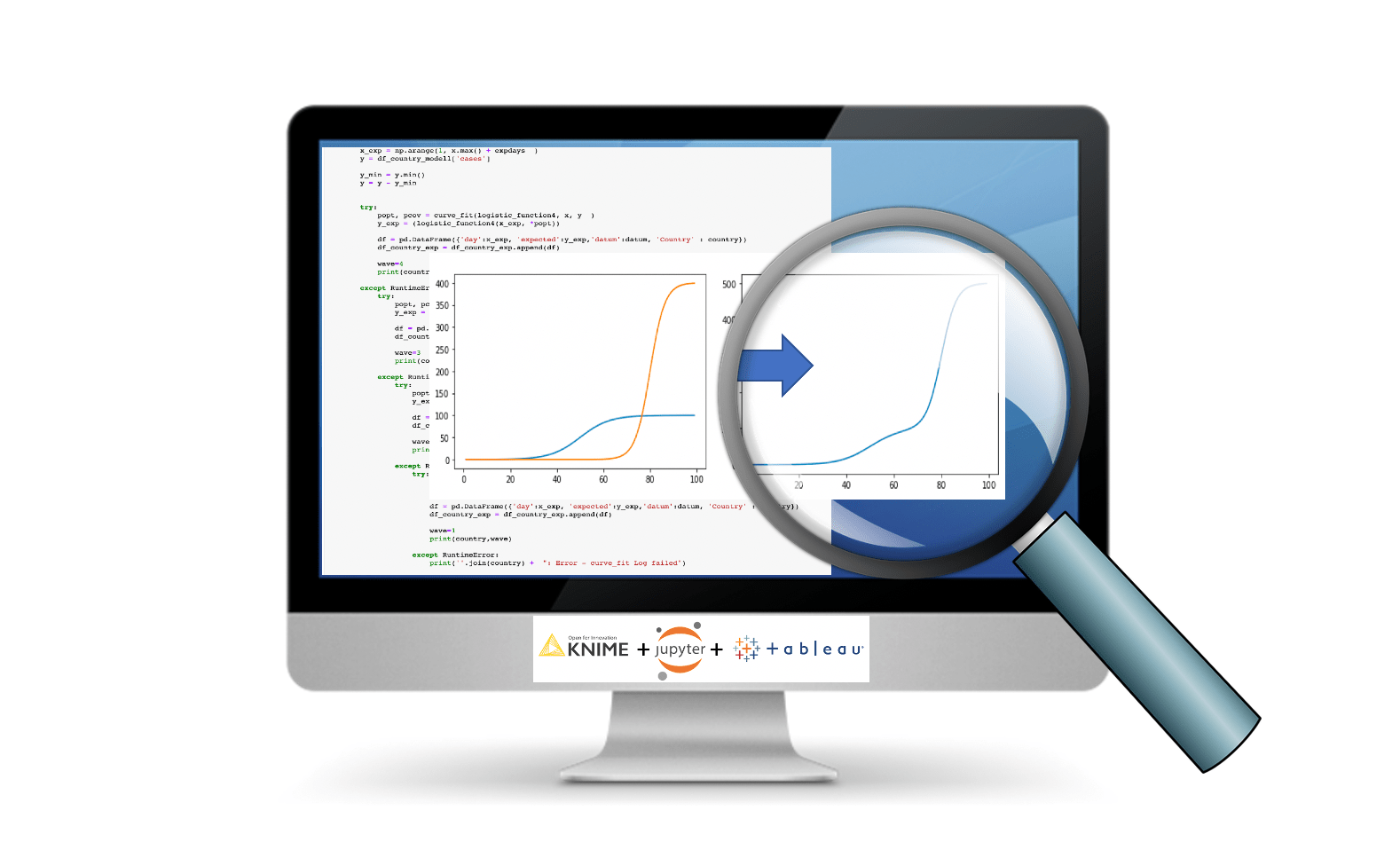In the last article we showed how to make a forecast for the next 30 days using covid data from the Johns Hopkins Institute with KNIME, Jupyter and Tableau. The projections were optimized for a logistic growth model. We will show that the decomposition of growth into S-shaped logistic components also known as Loglet analysis, is more accurate as it takes into account the evolution of multiple covid waves.

The term “Loglet”, coined at The Rockefeller University in 1994 joins “logistic” and “wavelet”. The Loglet analysis is interesting because it can handle multi-peak profiles which is a common challenge for curve fitting techniques.

The growth of replicating organisms, such as a virus, is basically characterized by three growth phases:

1. Exponential growth
2. Logistic growth
3. Multi-logistic growth

### 1. Exponential growth

In the first phase, growth is exponential. In mathematical terminology, the growth rate of a population P(t) is proportional to the population. The growth rate at time t is defined as the derivative dP(t)/dt .
The exponential growth model written as a differential equation is:Exponential growth model

This equation can be solved introducing e (the base of the natural logarithm, approximately 2.71 . . .).
The familiar solution is: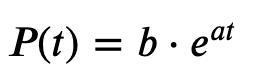where “a” is the growth rate constant and “b” is the initial population P(0). “a” is often expressed in percent. An “a” with a value of 0.02 is equivalent to the statement “the population was growing continuously at 2% per year” for example. Although many populations grow exponentially for a time, no finite system can sustain exponential growth indefinitely unless the parameters or limits of the system are changed.

Let’s try to visualize this growth in Jupyter-Notebooks:

``````import matplotlib.pyplot as plt
import numpy as np
import pandas as pdt = np.arange(1, 100)
a = 0.1
b = 1
y= b*np.exp(a*(t))

plt.plot(t,y)
plt.show()``````Exponential growth

### 2. Logistic growth

Since few systems sustain exponential growth over time, the exponential growth equation must be modified with a limit or carrying capacity that gives it the more realistic sigmoidal shape.

The most widely used modification of the exponential growth model is the logistic one. It was introduced by Verhulst in 1838, but popularized in mathematical biology by Lotka in the 1920s. The logistic equation begins with the exponential model but adds a “negative feedback” term that slows the growth rate of a population as the limit K is approached:Logistic differential equation

Notice that the feedback term (1−P (t))/K is close to 1 when P(t) << K and approaches zero as P(t) -> K. Thus, the growth rate begins exponentially but then decreases to zero as the population P(t) approaches the limit K, producing an S-shaped (sigmoidal) growth trajectory.

There are a number of closed form solutions to the logistic differential equation. We will use for our further analysis the Fisher-Pry equation:Logistic growth function

Equation above produces the familiar S-shaped curve. The three parameters are needed to fully specify the curve, “a”, “b”, and K. The growth rate parameter “a” specifies the “width” of the sigmoidal curve.

Let’s again to try to visualize this growth in Jupyter:

``````t = np.arange(0, 100)
K = 100
a = 0.1
b = 50

y = K/(1+np.exp(-a*(t-b)))

plt.plot(t,y)
plt.show()``````Logistic growth

Further it is often better to replace „α“ with a variable that specifies the time required for the curve to grow from 10% to 90% of the limit K, a period which we call characteristic duration Δt.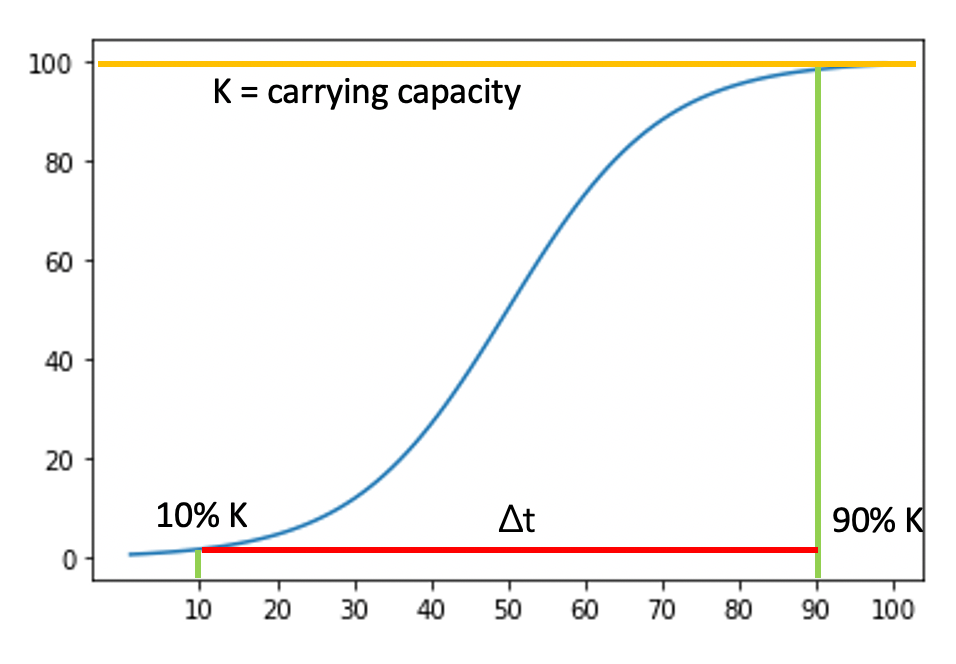Time required for the curve to grow from 10% to 90%

To achieve this, we have to perform the following algebraic transformations. First, we calculate the time needed to reach 90% of the total growth.Calculating time to reach 90% of the growth

Then the time to reach 10% of the total growth which we subtract from the value for 90%. The characteristic duration is related to „a“ by ln(81) / Δt.The parameter Δt is usually more useful than „a“ for the analysis of historical time-series data because the units are easier to appreciate. The three parameters K, ∆t, and „b“ define the parameterization of the logistic model used as the basic building block for our Loglet analysis.### 3. Multi-logistic growth

In the last article, we showed that the logistic growth model can be a good approximation for the evolution of a covid19 wave. But since this spread is not limited to a single wave, we need an extended approach to describe the entire process.

Many growth processes consist of several subprocesses. First, let us model a system that experiences growth in two discrete phases. Then, we will extend this model to an arbitrary number of phases. In the so called Bi-Logistic model, growth is the sum of two “wavelets”, each of which is logistic: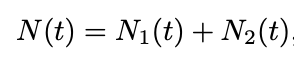where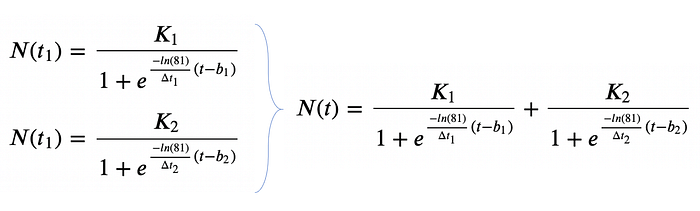The implementation of the Bi-Logistic model requires 6 parameters and is visualized in Jupyter as follows:

``````t = np.arange(1, 100)

K1 = 100
dt1 = 40
b1 = 50

K2 = 400
dt2 = 20
b2 = 80

y = K1/(1+np.exp(-np.log2(81)/dt1*(t-b1))) +
K2/(1+np.exp(-np.log2(81)/dt2*(t-b2)))

plt.plot(t,y)
plt.show()``````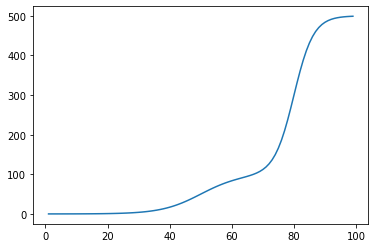Bi-Logistic growth

The next figure shows the decompositions of the Bi-Logistic wave. The blue pulse is superimposed by the orange pulse that follows. In this way, the seemingly complex behavior is reduced to the sum of the two logistic wavelets.

``````y1 = K1/(1+np.exp(-np.log2(81)/dt1*(t-b1)))
y2 = K2/(1+np.exp(-np.log2(81)/dt2*(t-b2)))

plt.plot(t,y1)
plt.plot(t,y2)
plt.show()``````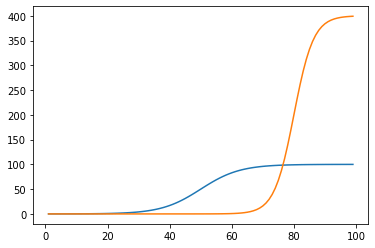The next step is a generalization of the Bi-Logistic model to a Multi-logistic model, where growth is the sum of n simple logistics: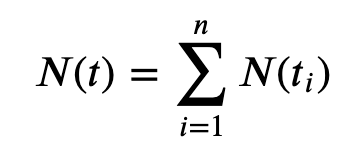where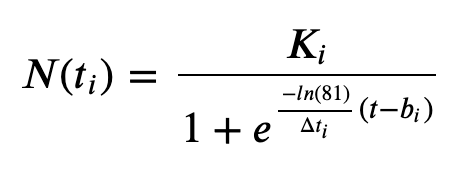Multi-logistic function

The following Jupyter code which is also available on my github-site, predicts for Switzerland the expected covid-19 cases for the next 30 days and requires the combination of 4 logistic wavelets. For other countries a different number of wavelets are necessary. We will come to this point below.

Fitting of the models was performed using the curve_fit function of the scipy package in Jupyter.

``````import matplotlib.pyplot as plt
import numpy as np
import pandas as pd
from scipy.optimize import curve_fitpath = 'https://raw.githubusercontent.com/deganza/Loglet-analysis-revisiting-covid19-projections/main/covid_daten_knime.csv'

df_country_list = df_country.groupby(['Country']).last().reset_index()
country_list = df_country_list['Country'].to_list()df_country_period = df_country
zeilen = df_country_period['cases'].count()# Select country or for evaluation of every country remark the variable country_list

#country_list =['Austria','Switzerland','Germany','United Kingdom','Spain']
#country_list =['United Kingdom']
#country_list =['Germany']
#country_list =['Spain']
country_list =['Switzerland']
#country_list =['Poland']
#country_list =['Turkey']
#country_list =['US']

df_country_exp = pd.DataFrame()

#logistic functions

def logistic_function1(x,b1,dt1,K1,b2,dt2,K2,b3,dt3,K3,b4,dt4,K4):
return K1/(1+np.exp(-np.log2(81)/dt1*(x-b1)))

def logistic_function2(x,b1,dt1,K1,b2,dt2,K2,b3,dt3,K3,b4,dt4,K4):
return  K1/(1+np.exp(-np.log2(81)/dt1*(x-b1))) + K2/(1+np.exp(-np.log2(81)/dt2*(x-b2)))

def logistic_function3(x,b1,dt1,K1,b2,dt2,K2,b3,dt3,K3,b4,dt4,K4):
return  K1/(1+np.exp(-np.log2(81)/dt1*(x-b1))) + K2/(1+np.exp(-np.log2(81)/dt2*(x-b2))) + K3/(1+np.exp(-np.log2(81)/dt3*(x-b3)))

def logistic_function4(x,b1,dt1,K1,b2,dt2,K2,b3,dt3,K3,b4,dt4,K4):
return  K1/(1+np.exp(-np.log2(81)/dt1*(x-b1))) + K2/(1+np.exp(-np.log2(81)/dt2*(x-b2))) + K3/(1+np.exp(-np.log2(81)/dt3*(x-b3))) + K4/(1+np.exp(-np.log2(81)/dt4*(x-b4)))

for country in country_list:

df_country_modell = df_country_period[df_country['Country'] == country]

expdays = 30
wave = 0

datum = pd.date_range(start=df_country_modell['Date'].max(), periods=expdays)
datum = pd.date_range(start=df_country_modell['Date'].min(),end=datum.max())
datum = datum.strftime("%Y-%m-%d")

zeilen= df_country_modell['cases'].count()
x = np.arange(1,zeilen+1)
x_exp = np.arange(1, x.max() + expdays  )
y = df_country_modell['cases']

y_min = y.min()
y = y - y_min

try:
popt, pcov = curve_fit(logistic_function4, x, y  )
y_exp = (logistic_function4(x_exp, *popt))

df = pd.DataFrame({'day':x_exp, 'expected':y_exp,'datum':datum, 'Country' : country})
df_country_exp = df_country_exp.append(df)

wave=4
print(country,wave)

except RuntimeError:
try:
popt, pcov = curve_fit(logistic_function3, x, y  )
y_exp = (logistic_function3(x_exp, *popt))

df = pd.DataFrame({'day':x_exp, 'expected':y_exp,'datum':datum, 'Country' : country})
df_country_exp = df_country_exp.append(df)

wave=3
print(country,wave)

except RuntimeError:
try:
popt, pcov = curve_fit(logistic_function2, x, y  )
y_exp = (logistic_function2(x_exp, *popt))

df = pd.DataFrame({'day':x_exp, 'expected':y_exp,'datum':datum, 'Country' : country})
df_country_exp = df_country_exp.append(df)

wave=2
print(country,wave)

except RuntimeError:
try:
popt, pcov = curve_fit(logistic_function1, x, y  )
y_exp = (logistic_function2(x_exp, *popt))

df = pd.DataFrame({'day':x_exp, 'expected':y_exp,'datum':datum, 'Country' : country})
df_country_exp = df_country_exp.append(df)

wave=1
print(country,wave)

except RuntimeError:
print(''.join(country) +  ": Error - curve_fit Log failed")

df_country_exp.reset_index(drop=True, inplace=True)
print('finished')
print(zeilen)``````

For every country, the model fits several logistic pulses and calculates the parameters which optimally describe the spread of the virus.

### How much training data is necessary?

We have now created a model that can approximate multiple consecutive and overlapping covid waves. But now the question rises: how many waves do we have to consider? This is a parameter that has not been calculated yet.

“Study the past if you would define the future.”
― Confucius

In the last article, we decided to consider only the cases of the last 90 days to fit our logistic model. With our new Multi-logistic model, this limitation is no longer necessary because we can use data from the first day of the pandemic outbreak. But now we have a new parameter to choose for the number of wavelets. This is an open point an we decide to take the following strategy:

It’s now Jan 2021. For every country we start to fit the model with 4 wavelets. If the model doesn’t converge, we reduce it to 3 wavelets and so on until we found the optimal approximation.

### Putting it all together

With the code above it’s already possible to make forecasts for every country, but we want to implement it in our existing KNIME-Workflow process for better data transformation and subsequent visualization in Tableau.

As you remember from the last article we use KNIME to load the covid19 data from the Johns Hopkins institute, transform it and calculate the forecasts with Jupyter. Finally we export the data in Tableau to visualize it.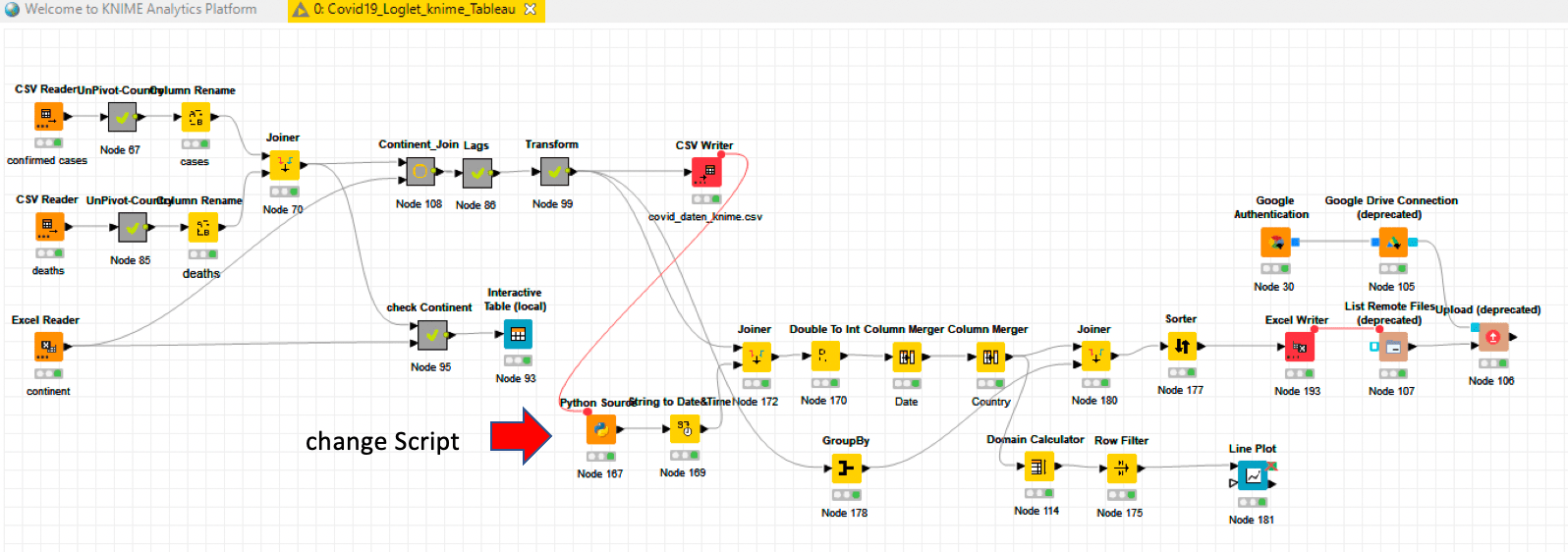KNIME Workflow: loads data, calculate forecasts and export it in Tableau

The KNIME-workflow is available on my KNIME-hub site and the Tableau Dashboard is published with the actual data nearly every day on my Tableau-Public site herecovid19 Tableau-Dashboard with projections for the next 30 days

This extended Loglet-prediction method is much more flexible than the simple logistic analysis because it takes into account the evolution of continuous waves. New waves or mutations of the virus can thus be tracked and extrapolated more quickly.

And that is becoming more and more important as this virus has already mutated into a more contagious version in the United Kingdom and in South Africa.

Material for this project

References